# Deriving the impedance equation of reactive passive components

Hi,
The origin of this question was contemplating how to express the impedance of an inductor as a function of frequency, for non sinusoidal voltage wave-forms such as triangle waves, but in particular rectangular pulse trains.

So going back to basics, I watched this video:

He derives the impedance of the inductor from v = L* di/dt
where i = ejwt
so v = L * d(ejwt)/dt
= jwL* ejwt

and so v/i = jwL

which I don't like because it seems like it is putting the cart before the horse, because you can apply a voltage across an inductor, but it's the current which is the dependent variable.

So I'd prefer to set v = ejwt so

i = 1/L * ∫ v dt

= 1/L * ∫ ejwt dt

= 1/L * 1/jw * ejwt + Constant

∴ v / i = jwL - Constant

My first question is, is there a reason why both methods are justified? I can see that the former is more simple because you don't have the 'constant'.

Okay, back to the main question of this post, taking for example a triangle wave as the current: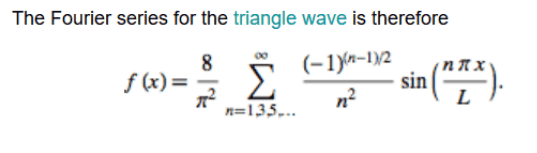So say I only went to n degree of 1 for simplicity. Then this would be:
V = Linductance * (d f(x)/dt)
V = Linductance* 8/Pi^2 * Pi/Fourier_L * cos (pi*x / Fourier_L)
so X_l = Linductance* 8/Pi^2 * Pi/Fourier_L * cos (pi*x / Fourier_L) / f(x)
which would be very complicated...

#### Attachments

Homework Helper
Gold Member
2020 Award
I think you are on the right track, but a difficulty arises with the complex impedance ## j \omega L ##, because the complex Fourier transform of the current waveform that you are using will have both real and imaginary parts. The periodic waveform will also result in delta functions of the fundamental frequency plus each the harmonics. What you are trying to do would most likely get treated in a course on Fourier analysis and/or linear response theory. ## \\ ## The voltage across the inductor is linearly related to the current in the inductor. Because the voltage leads the current, I think it might be necessary to write this linear relationship in the form ## I(t)=\int\limits_{-\infty}^{t} m(t-t') V(t') \, dt' ##. By the convolution theorem ## \tilde{I}(\omega)=\tilde{m}(\omega) \tilde{V}(\omega) ## where ## \tilde{I}(\omega)=\int\limits_{-\infty}^{+\infty} I(t)e^{-i \omega t} \, dt ##, and similarly for ## \tilde{m}(\omega) ## and ## \tilde{V}(\omega) ##. ## \\ ## A complete analysis should give that ## \tilde{m}(\omega) =\frac{1}{i \omega L } ##. ## \\ ## I have previously done an analysis like this on R-C circuits, and I think this one would proceed similarly. (A complete linear response analysis might require introducing a resistor in the circuit, and treating the case of an R-L circuit. That's really how I would proceed=what I showed you above might run into problems otherwise when you try to find the response of the inductor to a delta function voltage input). In any case though, I recommend you look for a good textbook on linear response theory, because you have kind of the right idea, but I think there are details that appear in the complex analysis that need to be included. ## \\ ## Note: I substituted ## i ## for ## j ## here.

•jim hardy and tim9000
I think you are on the right track, but a difficulty arises with the complex impedance ## j \omega L ##, because the complex Fourier transform of the current waveform that you are using will have both real and imaginary parts. The periodic waveform will also result in delta functions of the fundamental frequency plus each the harmonics. What you are trying to do would most likely get treated in a course on Fourier analysis and/or linear response theory. ## \\ ## The voltage across the inductor is linearly related to the current in the inductor. Because the voltage leads the current, I think it might be necessary to write this linear relationship in the form ## I(t)=\int\limits_{-\infty}^{t} m(t-t') V(t') \, dt' ##. By the convolution theorem ## \tilde{I}(\omega)=\tilde{m}(\omega) \tilde{V}(\omega) ## where ## \tilde{I}(\omega)=\int\limits_{-\infty}^{+\infty} I(t)e^{-i \omega t} \, dt ##, and similarly for ## \tilde{m}(\omega) ## and ## \tilde{V}(\omega) ##. ## \\ ## A complete analysis should give that ## \tilde{m}(\omega) =\frac{1}{i \omega L } ##. ## \\ ## I have previously done an analysis like this on R-C circuits, and I think this one would proceed similarly. (A complete linear response analysis might require introducing a resistor in the circuit, and treating the case of an R-L circuit. That's really how I would proceed=what I showed you above might run into problems otherwise when you try to find the response of the inductor to a delta function voltage input). In any case though, I recommend you look for a good textbook on linear response theory, because you have kind of the right idea, but I think there are details that appear in the complex analysis that need to be included. ## \\ ## Note: I substituted ## i ## for ## j ## here.
Hi Charles,
Great response. It's been too many years since I did Signals and Systems, it would probably take me a full day if I was to try and convolve something simple on paper. I should look into linear response theory when I can; I can't even really remember what a Bode plot is.
You point out something fundamental, which is that it would have real and imaginary parts, which I didn't contemplate.

So this all started when I was wondering what the impedance of an inductor would be at 250khz for an ON/OFF duty cycle. But I gather that the conveniences were are afforded with sinusoidal prevent people from determining such simple impedance values for non-sinusoidal supplies. That is a shame.

•Homework Helper
Gold Member
2020 Award
So this all started when I was wondering what the impedance of an inductor would be at 250khz for an ON/OFF duty cycle. But I gather that the conveniences were are afforded with sinusoidal prevent people from determining such simple impedance values for non-sinusoidal supplies. That is a shame.
The impedance is a function of the frequency component that is being considered, (as opposed to the impedance being constant and independent of frequency). The result is that the voltage waveform is also periodic, but it is a distorted version of the current waveform. A square-wave for the current will not result in a square-wave for the voltage.

Homework Helper
Gold Member
2020 Award
One additional item: I believe in the complex Fourier formulation, (which is often simplified for one particular frequency and starting with a voltage ## V(t)=V_o e^{i \omega t} ##, you finish up by simply looking at the real part), if you use it and operate on a square wave composed of Fourier components, if you want to know what is going on at a particular frequency ## \omega_o ##, you need to look at the composite of ## \omega_o ## and ## -\omega_o ##.

•tim9000
The impedance is a function of the frequency component that is being considered, (as opposed to the impedance being constant and independent of frequency). The result is that the voltage waveform is also periodic, but it is a distorted version of the current waveform. A square-wave for the current will not result in a square-wave for the voltage.
Yes, no argument there, I can imagine integrating a square wave gives a ramp function, and there is an integral relationship between magnetic flux (a proxy for current) and applied square voltage over time, etc.

One additional item: I believe in the complex Fourier formulation, (which is often simplified for one particular frequency and starting with a voltage ## V(t)=V_o e^{i \omega t} ##, you finish up by simply looking at the real part), if you use it and operate on a square wave composed of Fourier components, if you want to know what is going on at a particular frequency ## \omega_o ##, you need to look at the composite of ## \omega_o ## and ## -\omega_o ##.
To be honest, I don't know what to say here that won't incriminate myself as a dullard. Because the extent of my memory is: I remember fourier series is for repetitive signals, fourier transform is for non-periodic, I vaguely remember how to calculate the series coefficients, I remember this make odd and even functions important but little else. I remember seeing the spectral plot with the -wo side, but I do not remember the significance of what that tells us...

Homework Helper
Gold Member
2020 Award
The complete (and complex) representation also works for periodic signals. The F.T. of ## F(t)=e^{+i \omega_o t} ## is ## \tilde{F}(\omega)=\int\limits_{-\infty}^{+\infty} e^{i (\omega_o-\omega) t} \, dt=2 \pi \, \delta(\omega-\omega_o) ##. ## \\ ## For an arbitrary shaped periodic function, the result will be a summation of the amplitudes from the discrete Fourier transform multiplied by the delta functions of the fundamental frequency plus that of each of the harmonics. ## \\ ## It might interest you also that the inverse Fourier transform is ## F(t)=\frac{1}{2 \pi} \int\limits_{-\infty}^{+\infty} \tilde{F}(\omega)e^{i \omega t} \, d \omega ##. ## \\ ##Notice for the case of ## \tilde{F}(\omega)=2 \pi \, \delta(\omega-\omega_o) ##, we recover ## F(t)=e^{i \omega_o t} ## when we perform the inverse transform. ## \\ ## Meanwhile, the negative frequency components are necessary if you work with a completely real voltage input. e.g. ## \cos(\omega_o t)=\frac{e^{i \omega_o t}+e^{-i \omega_o t}}{2} ##. The alternative is to assume you just want to look at the real part of ## e^{i \omega_o t} ##, but that is not as mathematically complete as treating it as composed of both positive and negative frequencies.## \\ ## For an arbitrary function, (such as the square wave), it would be very clumsy to treat it as the real part of some collection of complex terms. It's easier just to compute it with both positive and negative frequencies of the form ## e^{i \omega t} ##.

Last edited:
Homework Helper
Gold Member
2020 Award

Hello.
$$\textbf{Notation and mathematics used}$$
This denotes the real part of the complex number:
$$\Re \Big[ e^{j(a)} \Big] = cos(a)$$
The property below is about how exponents are summed when they are multiplied by a common base, this allows one to remove the frequency aspect from the rotating phasor and express it as a complex number with magnitude and phase:
$$Ae^{j(\omega t + \phi)} = e^{j(\omega t)} \cdot A e^{j(\phi)}$$
An alternate way to express complex numbers in polar form:
$$A e^{j(\alpha)} = A \angle{\alpha}$$
$$\textbf{Sinusoidal steady state: Impedence}$$
Impedence is the complex number that captures the attenuation between voltage peak and current peak, and the phase difference. In electrical engineering, these peak values are RMS values, for sinusoids of the form:
$$A_{p} \cos( \omega t + \phi )$$
the RMS value is always:
$$\dfrac{A_{p}}{\sqrt{2} }$$
$$\textbf{Sinusoidal steady state: The phasor transform}$$
The operation that extracts, from a sinusoid, a complex number that captures the:
1. The RMS magnitude of that sinusoid.
2. The phase shift of that sinusoid.
$$A_{p} \cos( \omega t + \phi )\,\,\,\, \text{V} = \Re \Big[ A_{p} e^{j( \omega t + \phi) } \Big] = \Re \Big[ e^{j( \omega t) } \cdot \underline{A_{p}e^{j(\phi)}} \Big] \rightarrow \dfrac{A_{p}}{\sqrt{2}} e^{j(\phi )} \,\,\,\, \text{V}$$
The complex number that captures the phase and RMS magnitude of the sinusoid: ##A_{p} \cos( \omega t + \phi )\,\,\,\, \text{V} ## is:

$$\dfrac{A_{p}}{\sqrt{2}} e^{j(\phi )} \,\,\,\, \text{V} = \dfrac{A_{p}}{\sqrt{2}} \angle{ \phi} \,\,\, \text{V}$$
The phasor is independent of the frequency, as you saw above we extracted the frequency out of the complex phasor. The limitation of phasor analysis is it relies on circuits where the frequencies of the sources are all of the same. If the sinusoids are not at the same frequency then we would have to use superposition. Impedence is the division of the complex numbers that are representing the voltage and current.
$$Z = X \angle{ \phi } \,\,\,\,\,\, \Omega= \dfrac{ \dfrac{V_{p} }{\sqrt{2} } \angle{ \phi_{1} } \,\,\,\, \text{V} }{ \dfrac{A_{p} }{ \sqrt{2} } \angle{\phi_{2} } \,\,\,\, \text{A} }$$
$$\textbf{Derivatives and integral of phasors}$$
In the time domain, differentiation of a sinusoid is equivalent to multiplication of its phasors by ## j \omega ##. Time integration, is equivalent to the multiplication of its phasor by ## \dfrac{1}{ j \omega} ##

$$\dfrac{ d^{n}}{d^{n}t} A_{p} \cos( \omega t + \phi) \,\,\,\, \text{V} \iff { (j \omega)}^{n} \dfrac{A_{p}}{\sqrt{2}} \angle{ \phi}\,\,\,\, \text{V}$$
$$\displaystyle \int A_{p} \cos( \omega t + \phi) \,\, \,\, \text{V} \,\,\, \text{dt} \iff \dfrac{1}{j \omega} \dfrac{A_{p}}{\sqrt{2}} \angle{ \phi} \,\,\,\, \text{V}$$

To derive the impedence for R, L, and C, we use their simple voltage and current relationships, and assume we are forcing a voltage function of the form:
## v(t) = V_{p} \cos(\omega t) ##
$$I_{C}(t) = Cv'(t) \,\,\,\,\,\,\,\,\, I_{L}(t) = \dfrac{1}{L} \displaystyle \int_{-\infty}^{t} v(t) \,\,\,\,\, \text{dt}$$

Using what we have gathered from the above sections:
$$V_{c}(t) =\Re \Big[ A_{p} e^{j( \omega t ) } \Big] \rightarrow \dfrac{A_{p}}{\sqrt{2}} \angle{0} \,\,\,\,\text{V} \,\,\,\,\, I_{c}(t) = C \cdot \dfrac{d}{dt} \Re \Big[ A_{p} e^{j( \omega t ) } \Big] \rightarrow ?$$

$$I_{c}(t) = C \cdot \dfrac{d}{dt} \Re \Big[ A_{p} e^{j( \omega t ) } \Big] = j \omega C \Re \Big[ A_{p} e^{j( \omega t ) } \Big] \rightarrow j \dfrac{A_{p}\omega C}{\sqrt{2}} \angle{0} = e^{j(\frac{\pi}{2})} \cdot \dfrac{A_{p}\omega C}{\sqrt{2}} \angle{0} = \dfrac{A_{p}\omega C}{\sqrt{2}} \angle{\frac{\pi}{2} } \,\,\,\, \text{A}$$

The impdence, is the ratio of these two complex numbers:
$$Z_{c} = \dfrac{\dfrac{A_{p}}{\sqrt{2}} \angle{0} \,\,\,\,\text{V} }{ \dfrac{A_{p}\omega C}{\sqrt{2}} \angle{\frac{\pi}{2} } \,\,\,\, \text{A}} =\dfrac{A_{p}}{\sqrt{2}} \cdot \dfrac{ \sqrt{2} }{ A_{p} \omega C} \angle{0 - \frac{\pi}{2} } = \dfrac{1}{ \omega C} \angle{ - \frac{\pi}{2} } = - j \dfrac{1}{\omega C} \,\,\,\,\, \Omega$$
The impedence for the inductor can also be derived in the same manner. Sinusoidal steady state impedence analysis requires voltage and current to be sinusoids. The method for deriving impedences is this:
1. Force a voltage function which is a sinusoid of the form ## A_{p} \cos(\omega t + \phi) ##
2. Solve for the complex number that represents the current for the inductor.
3. Divide voltage phasor (the complex number) by current phasor, and you will get your frequency dependent impedence function for the inductor.

$$\textbf{Fourier series and linear circuits: Circuits driven by non sinusoidal periodic waveforms}$$
Since the impedence and phasor transform methods apply only for pure sinusoids, the fourier series is useful to analyse circuits which are affected upon by non sinusoidal functions, which can be transformed to a fourier series representation, we prefer to use the amplitude phase format of the fourier series.
1. The first step is to express the excitation ## f(t) ## as a fourier series.
2. Transform the circuit from the time domain to the frequency (phasor) domain.
3. Find the response to DC (zero frequency or mean value of your fourier series) and then find the responses to all the AC components.
4. Use superposition to sum up all DC and AC responses, adding them up.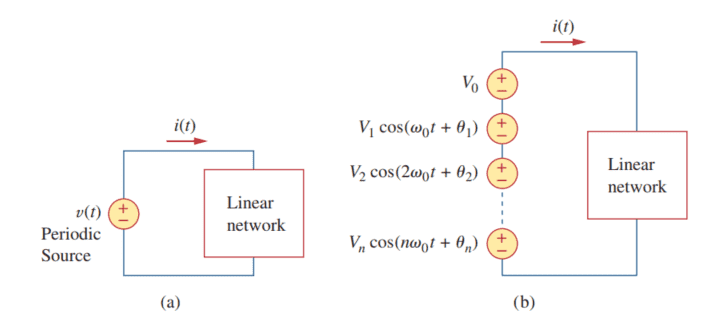$$\textbf{Amplitude phase format of the fourier series}$$

$$f(t) = \dfrac{ a_{0}}{2} + \displaystyle \sum_{n=1}^{n \to \infty} \Big|\textbf{A}_{n} \Big| \cos( n \omega_{0} t - \phi )$$ Where $$\,\,\,\,\,\, \Big|\textbf{A}_{n} \Big| = \sqrt{a_{n}^2 + b_{n}^{2} } \,\,\,\,\,\, \phi = \arctan{ \Big( \dfrac{b_{n}}{a_{n}} \Big)}$$

$$\textbf{Easy example demonstrating use of superposition}$$
You have a single capacitor of $$22 \mu \text{F}$$ acted upon by three sources:

$$v_{0}(t) = 1 \,\,\,\, \text{V} \,\,\,\,\,\,\,\,\, v_{1}(t) = 5 \cos( 10t + 20) \text{V} \,\,\,\,\,\,\,\,\, v_{2}(t) = 10 \cos(20t + 30)$$
We will have to use superposition for three currents:
$$I_{0} = 0 \,\,\,\,\, I_{1} = \dfrac{ \dfrac{5}{\sqrt{2} } \angle{20} }{\dfrac{1}{10 \cdot 22 \mu \text{F} } \angle{90} } \,\,\,\, \text{A} = 777.8 \angle{-70} \,\,\,\,\, \mu \text{A} \,\,\,\,\,\,\, I_{2} = \dfrac{ \dfrac{10}{\sqrt{2}} \angle{30} }{ \dfrac{1}{ 20 \cdot 22 \mu \text{F} } \angle{90} } = 3110 \angle{-60} \,\,\,\, \mu \text{A}$$
These are the phasors that represent current for the DC, first harmonic, and third harmonic, they cannot be added up as they arise from different frequencies. In the time domain, however, they can be summed up as a single current function:
$$i(t) = 777.8 \cos(10t -70) + 3110 \cos(20t -60) \,\,\,\,\,\,\, \mu \text{A}$$
Inserting a waveform as expressed as a fourier series:
$$v(t) = \textbf{V}_{0} + \displaystyle \sum_{n=1}^{n \to \infty} \Big|\textbf{V}_{n} \Big| \cos(n\omega_{0} t + \phi_{1})$$
$$i(t) = \textbf{I}_{0} + \displaystyle \sum_{n=1}^{n \to \infty} \Big|\textbf{I}_{n} \Big| \cos(n\omega_{0} t + \phi_{2})$$
This is due to the superposition that arises in linear circuits.

#### Attachments

Last edited:
Hello.

$$\textbf{Example: RL circuit forced upon by a unipolar square wave with half duty cycle}$$
I just derived this easy example myself using this setup:
$$R = 10 \,\,\,\, \Omega \,\,\,\,\,\,\,\,\,\,\,\,\,\,\, L = 2 \,\, \text{H}$$
The wave here is:
$$v(t) = \begin{cases} U_{0} & \text{ t \in [ 0 , \frac{T_{0}}{2} ]} \\ 0 & \text{ t \in [\frac{T_{0}}{2}, T_{0} ] } \\ \end{cases}$$
Periodic with ##T_{0}##. and thus the fundamental frequency is: ## \omega_{0} = \dfrac{2 \pi}{T_{0}} ##
We first derive the expression for the voltage across the inductance, using phasors:
$$V_{L} = V_{s} \cdot \dfrac{ j \omega L }{ R + j \omega L } = V_{s} \cdot \dfrac{j 2 \omega}{10 + j 2 \omega}$$

$$V_{L} = V_{s} \cdot \dfrac{j 2 \omega}{10 + j 2 \omega}$$
Now after deriving the fourier transform I got:
$$v(t) = \dfrac{U_{0}}{2} + \displaystyle \sum_{n = 1, 3, 5, 7, 9..}^{n \to \infty} \dfrac{2 U_{0}}{n \pi} \sin(n \frac{2 \pi}{T_{0}} t)$$
Now finding the responses for all the harmonics, with ## n \omega_{0} ## and converting the sine in the fourier transform to its phasor:
$$\dfrac{2 U_{0}}{n \pi} \sin(n \frac{2 \pi}{T_{0}} t) \rightarrow - \dfrac{2 U_{0}}{n \pi}j$$
Plugging this into the impedence voltage divider for the inductor and multiplying by the admittance of the inductor, to find the current, the impedence frequency expression for all harmonics becomes:
$$- \dfrac{2 U_{0}}{n \pi}j \cdot \dfrac{j 2 n \omega_{0} }{10 + j 2 n \omega_{0} } \cdot \dfrac{1}{j 2 n \omega_{0} } = - \dfrac{2 U_{0}}{n \pi}j \cdot \dfrac{1}{ 10 + j 2 n \omega_{0} }$$
We know the phasor current for the inductor at all odd number multiples of the fundamental frequency now:

$$I_{L (n \omega_{0})} = - \dfrac{2 U_{0}}{n \pi}j \cdot \dfrac{1}{ 10 + j 2 n \omega_{0} }$$
Expressing the above as a complex number with magnitude and phase:
$$I_{L (n \omega_{0})} = \dfrac{ 2 U_{0} }{ n \pi \sqrt{100 + 4n^{2} {\omega_{0}}^{2} } } \angle{ - \dfrac{\pi}{2} - \arctan{\Big[ \dfrac{n \omega_{0}}{5} \Big]} }$$
Converting this to the time domain:

$$i(t, n \omega_{0} ) = \dfrac{ 2 U_{0} }{ n \pi \sqrt{100 + 4n^{2} {\frac{2 \pi}{T_{0}} }^{2} } } \cdot \sin \Bigg[n \frac{2 \pi}{T_{0}} t - \arctan{\Big[ \dfrac{n \omega_{0}}{5} \Big]} \Bigg]$$
The fourier series for the current is:
$$i(t) = \dfrac{U_{0}}{20} + \large \displaystyle \sum_{n = 1, 3, 5,.. }^{n \to \infty} \dfrac{ 2 U_{0} }{ n \pi \sqrt{100 + 4n^{2} {\left(\frac{2 \pi}{T_{0}} \right)}^{2} } } \cdot \sin \Bigg(n \frac{2 \pi}{T_{0}} t - \arctan{\Big[ \dfrac{n \omega_{0}}{5} \Big]} \Bigg) \,\,\,\,\, \text{A}$$
So its not that complicated.
The input waveform is expressed as a fourier series.
The output relation with the input is found using physical laws or circuit theorems in the frequency phasor domain.
The input is expressed as a phasor, and then plugged into the relation of the frequency domain, the dependence on the harmonics is also considered.
This result is then converted back to the time domain, and expressed as another fourier series.

Last edited:
•jim hardy
Gold Member
Dearly Missed
es, no argument there, I can imagine integrating a square wave gives a ramp function, and there is an integral relationship between magnetic flux (a proxy for current) and applied square voltage over time, etc.

here's a real 'scope trace of current through primary(top trace) and voltage induced in secondary(bottom trace) of a twelve foot tall dual winding inductor from my power plant.
It shows the basic triangle-square derivative/integral relation clearly, i think.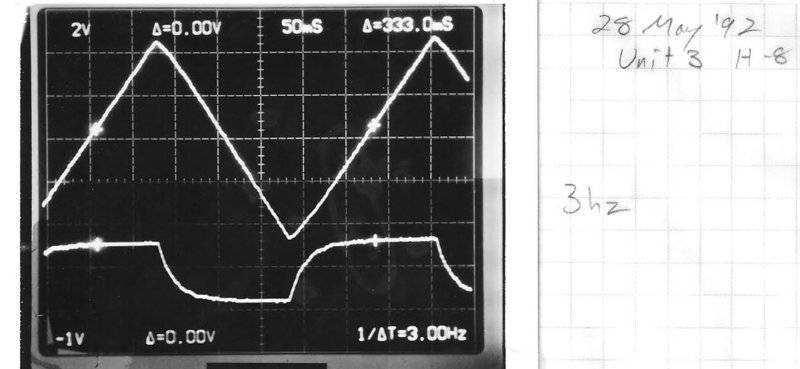Those rounded corners on the induced voltage we attributed to eddy currents in the non-laminated core.

old jim

#### Attachments

•Hi.
I provided a method up top, but:
Here is an easy way to do it, using the laplace transform, and its generalisation the fourier transform:
$$\textbf{Deriving the impedence of an inductor using the laplace and fourier transform}$$

$$i_{L}(t)= \dfrac{1}{L} \cdot \displaystyle \int v(t) \,\,\,\,\, \text{dt}$$
Use the laplace transform property that:
$$\displaystyle \int f(t) \,\,\,\, \text{dt} \iff \dfrac{F(s)}{s}$$
Simply find the laplace transform and take the ratio of voltage over current:
$$I(s) = \dfrac{V(s) }{sL} \iff \dfrac{V(s)}{I(s)} = sL$$
When ## s = j \omega ## we generalised the laplace to the fourier transform, and thus:
$$\dfrac{V(j \omega)}{I(j \omega)} = j \omega L$$
$$Z{(j \omega)} = j \omega L \,\,\,\,\, \Omega$$
The region of convergence of the laplace transform must include the ## j \omega ## axis for this fourier transform to exist, and it does for R,L,C components.
$$\textbf{Deriving the impedence of a capacitor using the laplace and fourier transform}$$

$$i_{c}(t) = C \cdot v'(t)$$
Use the first derivative property of the laplace transform:
$$f'(t) \iff sF(s) - f(0^{-})$$
Since this is steady state, we assume no initial conditions, or i think the assumption is that there were initial conditions but now they have decayed or grown to the pure steady state bounds.
$$I(s) = sC V(s) \iff \dfrac{V(s)}{I(s)} = \dfrac{1}{sC}$$
When ## s = j \omega ## we generalised the laplace to the fourier transform, and thus:
$$\dfrac{V(s)}{I(s)} = \dfrac{1}{j \omega C}$$
$$Z(j \omega) = \dfrac{1}{j \omega C} \,\,\,\,\, \Omega$$
The fourier transform of some relation of a circuit is the same as taking the impedence definitions, the laplace transform is used when there are signals that the fourier transform cant handle, for many technical reasons.. anyway:
$$\textbf{Summary}$$
1. Use the laplace transform and its time derivatives and integral properties.
2. Find $$\dfrac{V(s)}{I(s)} = F(s)$$ and set ## s = j \omega ## and you have your frequency dependent impedance of the element.

Last edited:
•tim9000
Hello.

$$\textbf{Example: RL circuit forced upon by a unipolar square wave with half duty cycle}$$
I just derived this easy example myself using this setup:
$$R = 10 \,\,\,\, \Omega \,\,\,\,\,\,\,\,\,\,\,\,\,\,\, L = 2 \,\, \text{H}$$
The wave here is:
$$v(t) = \begin{cases} U_{0} & \text{ t \in [ 0 , \frac{T_{0}}{2} ]} \\ 0 & \text{ t \in [\frac{T_{0}}{2}, T_{0} ] } \\ \end{cases}$$
Periodic with ##T_{0}##. and thus the fundamental frequency is: ## \omega_{0} = \dfrac{2 \pi}{T_{0}} ##
We first derive the expression for the voltage across the inductance, using phasors:
$$V_{L} = V_{s} \cdot \dfrac{ j \omega L }{ R + j \omega L } = V_{s} \cdot \dfrac{j 2 \omega}{10 + j 2 \omega}$$

$$V_{L} = V_{s} \cdot \dfrac{j 2 \omega}{10 + j 2 \omega}$$
Now after deriving the fourier transform I got:
$$v(t) = \dfrac{U_{0}}{2} + \displaystyle \sum_{n = 1, 3, 5, 7, 9..}^{n \to \infty} \dfrac{2 U_{0}}{n \pi} \sin(n \frac{2 \pi}{T_{0}} t)$$
Now finding the responses for all the harmonics, with ## n \omega_{0} ## and converting the sine in the fourier transform to its phasor:
$$\dfrac{2 U_{0}}{n \pi} \sin(n \frac{2 \pi}{T_{0}} t) \rightarrow - \dfrac{2 U_{0}}{n \pi}j$$
Plugging this into the impedence voltage divider for the inductor and multiplying by the admittance of the inductor, to find the current, the impedence frequency expression for all harmonics becomes:
$$- \dfrac{2 U_{0}}{n \pi}j \cdot \dfrac{j 2 n \omega_{0} }{10 + j 2 n \omega_{0} } \cdot \dfrac{1}{j 2 n \omega_{0} } = - \dfrac{2 U_{0}}{n \pi}j \cdot \dfrac{1}{ 10 + j 2 n \omega_{0} }$$
We know the phasor current for the inductor at all odd number multiples of the fundamental frequency now:

$$I_{L (n \omega_{0})} = - \dfrac{2 U_{0}}{n \pi}j \cdot \dfrac{1}{ 10 + j 2 n \omega_{0} }$$
Expressing the above as a complex number with magnitude and phase:
$$I_{L (n \omega_{0})} = \dfrac{ 2 U_{0} }{ n \pi \sqrt{100 + 4n^{2} {\omega_{0}}^{2} } } \angle{ - \dfrac{\pi}{2} - \arctan{\Big[ \dfrac{n \omega_{0}}{5} \Big]} }$$
Converting this to the time domain:

$$i(t, n \omega_{0} ) = \dfrac{ 2 U_{0} }{ n \pi \sqrt{100 + 4n^{2} {\frac{2 \pi}{T_{0}} }^{2} } } \cdot \sin \Bigg[n \frac{2 \pi}{T_{0}} t - \arctan{\Big[ \dfrac{n \omega_{0}}{5} \Big]} \Bigg]$$
The fourier series for the current is:
$$i(t) = \dfrac{U_{0}}{20} + \large \displaystyle \sum_{n = 1, 3, 5,.. }^{n \to \infty} \dfrac{ 2 U_{0} }{ n \pi \sqrt{100 + 4n^{2} {\left(\frac{2 \pi}{T_{0}} \right)}^{2} } } \cdot \sin \Bigg(n \frac{2 \pi}{T_{0}} t - \arctan{\Big[ \dfrac{n \omega_{0}}{5} \Big]} \Bigg) \,\,\,\,\, \text{A}$$
So its not that complicated.
The input waveform is expressed as a fourier series.
The output relation with the input is found using physical laws or circuit theorems in the frequency phasor domain.
The input is expressed as a phasor, and then plugged into the relation of the frequency domain, the dependence on the harmonics is also considered.
This result is then converted back to the time domain, and expressed as another fourier series.

I am not up to date with this thread, but I had a spare 5 minutes and my eyes fell on your post. Wow, you say this isn't too complicated (and you are correct in the sense that what you have written is easy to follow) but I disagree in that it would have taken me a week to come up with what you just wrote. I really like it, good job.

I need to re-read this whole thread, and parts of it several times over, but am I interpreting the theoretical basis for your working that: since anything is comprised of an infinite amount of sineusoids (i.e. superposition) (or can be shown to be as such), the jwL relationship is still applicable for inductive reactance?

Thus you can just use the fundamental frequency and the rest of the harmonics in the Fourier transform?

That is really clever.

Last edited:
•AVBs2Systems
tech99
Gold Member
I think we can define impedance of any component to any waveform, but reactance is defined for a single frequency and so applies only to a sine wave or to one sinusoidal component of a complex wave.

I think we can define impedance of any component to any waveform, but reactance is defined for a single frequency and so applies only to a sine wave or to one sinusoidal component of a complex wave.
I haven't read any more of this thread other than your post, but I agree, it is for a single frequency in the same way that jwL is a simple function of w.
So I assume that if I divide: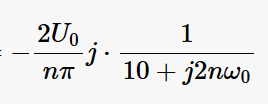by: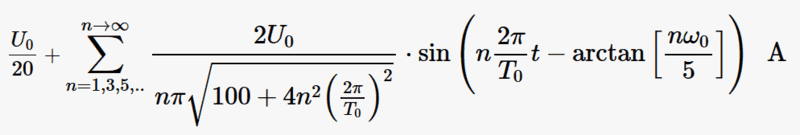to get V/I, it would also be a function of 'w' in the same way, with the small difference being w = wo.

But I'm just thinking out loud.

#### Attachments

•AVBs2Systems
Hi Tim.
I am sorry if I caused any confusion, I know it is not easy if you have been out of it for some time.

I haven't read any more of this thread other than your post, but I agree, it is for a single frequency in the same way that jwL is a simple function of w.
So I assume that if I divide:
View attachment 238670
by:
View attachment 238671
to get V/I, it would also be a function of 'w' in the same way, with the small difference being w = wo.

But I'm just thinking out loud.
if you simply divide that complex number by the complex number for current, you would get the impedence of the inductor, as we derived the current using the impedence voltage divider into the admittance. We had already assumed impedences when deriving the relation for current:
$$j n \omega_{0} L =\dfrac{V_{s} \cdot \dfrac{j n \omega_{0} L}{ 10 + j n \omega_{0} L } }{ I_{l} }$$
$$\textbf{The fourier series is used to express non sinusoidal periodic excitations as sinusoids, to allow for use of phasors}$$
The point of the fourier series in circuit analysis, is to simply find the output when the input is non sinusoidal. Not to derive impedences, because impedences are already assumed if youre using the fourier series.

The fourier series enables one to express the non sinusoidal excitation as a sinusoidal series summed up with a DC offset, the sinusoidal series can be converted to a complex number representation with its dependence on the harmonics ## n \omega_{0} ##, and then and only then plugged into the frequency dependent impedence relation.

All sinusoids can be represented in their magnitude and phase, by a complex number. This complex number captures the magnitude and phase of the sinusoid, but is not equal to it. This mapping is invertible, meaning every complex number also maps to a sinusoid with the same magnitude and phase.

After this, this frequency dependent impedence relation is converted back to the time domain with its dependence on ## n \omega_{0} ##.into another fourier series. You start off by knowing what the impedence relation is already, that could be a voltage divider or current, or something more complicated, and then convert the non sinusoidal excitation into a fourier series, this allows you to transform the function into complex numbers which are then used in the phasor domain, after this the result is converted back to the time domain, and due to superposition of linear electric circuits in the time domain, the result is another fourier series.
I am not up to date with this thread, but I had a spare 5 minutes and my eyes fell on your post. Wow, you say this isn't too complicated (and you are correct in the sense that what you have written is easy to follow) but I disagree in that it would have taken me a week to come up with what you just wrote. I really like it, good job.

I need to re-read this whole thread, and parts of it several times over, but am I interpreting the theoretical basis for your working that: since anything is comprised of an infinite amount of sineusoids (i.e. superposition) (or can be shown to be as such), the jwL relationship is still applicable for inductive reactance?

Thus you can just use the fundamental frequency and the rest of the harmonics in the Fourier transform?

That is really clever.
Yes, I think you understood my post, but best way to know is to do a few easy problems yourself.
And thank you! You're welcome.

Last edited:
jim hardy
Gold Member
Dearly Missed
..for non sinusoidal voltage wave-forms such as triangle waves, but in particular rectangular pulse trains .........

.........

So I'd prefer to set v = ejwt so

i = 1/L * ∫ v dt

= 1/L * ∫ ejwt dt

= 1/L * 1/jw * ejwt + Constant

∴ v / i = jwL - Constan

interesting.

A square wave might be represented by V(t) =A X sgn(sin(ωt))
and a triangle wave by the integral of that..
While i'm not sure signum function is integrable
it just might have a Fourier transform
http://www.thefouriertransform.com/pairs/step.php
says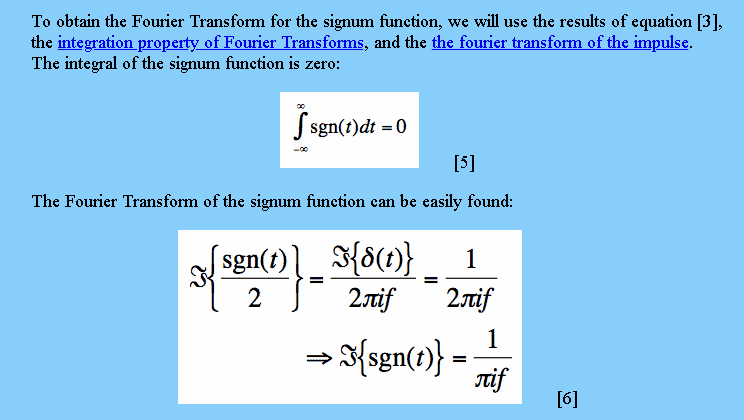and i know my limits.

old jim

Last edited: